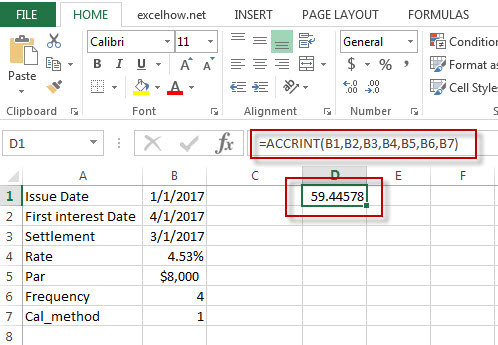# Excel Accrint Function

This post will guide you how to use Excel ACCRINT function with syntax and examples in Microsoft excel.

### Description

The Excel ACCRINT function returns the accrued interest for a security that pays periodic interest.

The ACCRINT function is a build-in function in Microsoft Excel and it is categorized as a Financial Function.

The ACCRINT function is available in Excel 2016, Excel 2013, Excel 2010, Excel 2007,  Excel 2011 for Mac.

### Syntax

The syntax of the ACCRINT function is as below:

`= ACCRINT (issue, first_interest, settlement, rate, par, frequency, [basis], [calc_method])`

Where the ACCRINT function arguments are:

• Issue -This is a required argument. The security’s issue date.
• First_interest – This is a required argument.  The security’s first interest date.
• Sattlement – This is a required argument.  The security’s settlement date.
• Rate – This is a required argument.  The security’s annual coupon rate.
• Par – This is a required argument.  The par value of the security. Note: If Par is omitted, the default Par value will be set to \$1000.
• Frequency – This is a required argument.  The frequency of the interest payments for the security. The following values can be set:
 1 Annual payments 2 Semiannual payments 4 Quarterly
•
Basis – This is an optional argument.  The type of day count basis to use. The following values can be set:
 Basis Day count basis 0 or omitted US (NASD) 30/360 1 Actual/actual 2 Actual/360 3 Actual/365 4 European 30/360
• Calc_method – This is an optional argument.  it can be set as the following values:
 0 Returns the accrued interest from first interest date  to settlement date 1 Returns the accrued interest from issue_date to settlement_date

### Excel ACCRINT Function Examples

The below examples will show you how to use Excel ACCRINT Function to return the accrued interest for a security that pays periodic interest.

`#1 =ACCRINT(B1,B2,B3,B4,B5,B6,B7)`### Related Functions

• Excel AccrintM Function
The Excel ACCRINTM function returns the accrued interest for a security that pays interest at maturity.The syntax of the ACCRINTM function is as below:= ACCRINTM(issue, settlement, rate, par, [basis])…